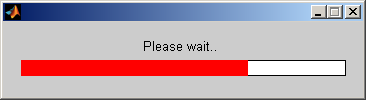# Matlab : Create Progressbar

When we process a looping function in Matlab, we can create progressbar in this function. Matlab have waitbar function. We can use this function if we want to add progressbar in my function. This is sample of picture progressbar in Matlab with function waitbar:This is example how to create progressbar in Matlab. Copy this code in your m-file editor and running this code.

```clear all;  %clear old data in workspace

itr = 100000; %number of iteration process

vblock = 100; %update progressbar every vblock
k = 1;
for i=1:itr  %loop data
if(k*vblock==i) %update progressbar
waitbar(i/itr);   %setting progressbar
k = k+1;
end
% PROCESS FUNCTION

end
close(h) %close progressbar```

This progressbar will updated every 100 data. This progressbar will caused program slower than without progressbar. If we want create progressbar without cause running function slower than without progressbar, we can add spesific value in number of update progressbar.  Example. When running a function, progressbar will updated every 10% of total data. We can modified above code with this :

```clear all;  %clear old data in workspace

itr = 100000; %number of iteration process

vblock = round(itr/10); %update progressbar every vblock
k = 1;
for i=1:itr  %loop data
if(k*vblock==i) %update progressbar
waitbar(i/itr);   %setting progressbar
k = k+1;
end
% PROCESS FUNCTION

end
close(h) %close progressbar```
1.2.